Next: BCS Theory for Conventional Superconductors Up: Magnetic Properties of Conventional Superconductors Previous: Ginzburg-Landau Theory

## The Clean and Dirty Limits

The purity of a superconductor is characterized by the ratio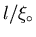.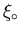is the coherence length of the pure material, and is given by Eq. (2.4). l is the electron mean free path, defined as: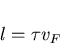(9)

where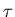is the time interval between collisions of conduction electrons with impurities in the sample. The magnitude ofis determined in the normal state. A sample is clean if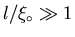, and dirty if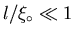. The actual coherence length when impurities are considered, is dependent upon the mean free path l. Intuitively, one can define an effective coherence length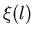such that [13,17]: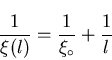(10)

According to Eq. (2.10), the coherence lengthbecomes shorter with decreasing l, so that in the dirty limit: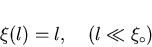(11)

and in the clean limit: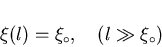(12)

Eq. (2.11) and Eq. (2.12) are valid only for T=0K. In the clean limit (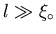) at T=0K, the magnetic penetration depth is the London penetration depth given in Eq. (2.1). The actual coherence length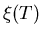and the observed penetration depth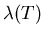as determined by microscopic theory are given in the clean limit () as :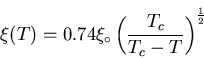(13)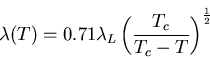(14)

and in the dirty limit (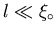):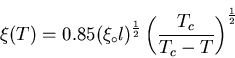(15)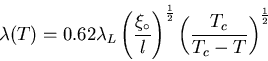(16)

where Equations (2.13) through (2.16) are valid only in the neighborhood of Tc, such that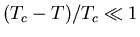. The important result is that according to Eqs.(2.15) and (2.16), as l decreases (i.e. the superconductor becomes more impure),increases, whiledecreases. Thus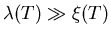at all temperatures in an impure material. The high-temperature superconductors have short coherence lengths on the order of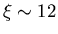to 15Å. Since the electron mean free path l is typically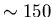Å in these materials, then they are well within the clean limit .Next: BCS Theory for Conventional Superconductors Up: Magnetic Properties of Conventional Superconductors Previous: Ginzburg-Landau Theory
Jess H. Brewer
2001-09-28